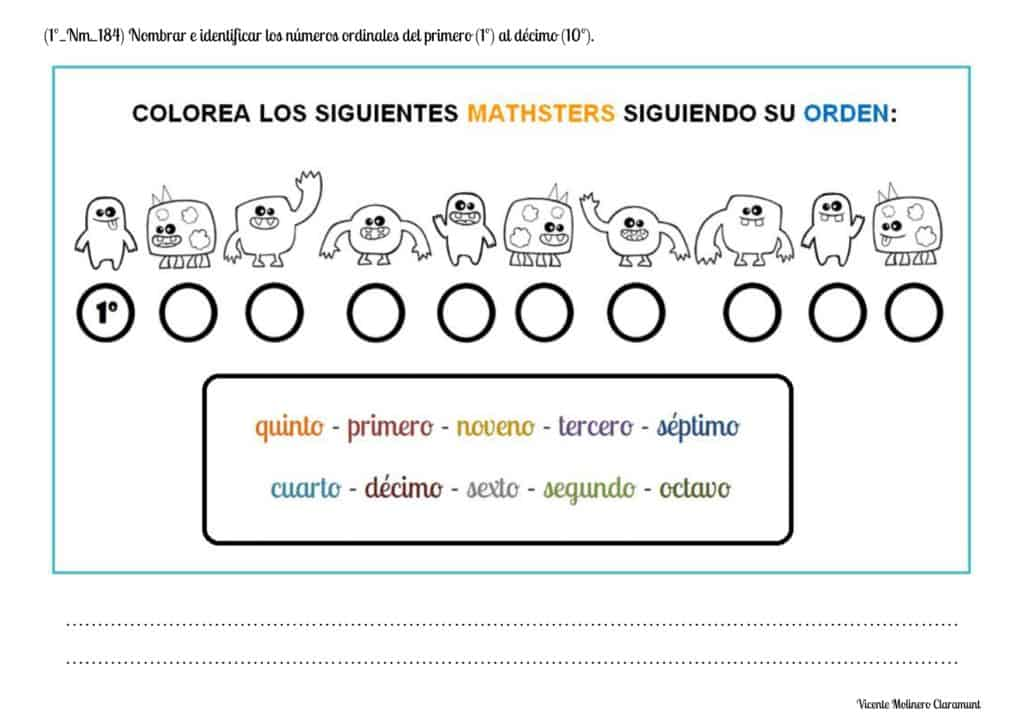# Ordinal Numbers

## NUMBERS AND OPERATIONSAn ordinal number is one that indicates the position or order that an element occupies in a set.

The cardinal numbers (1, 2, 3) are used to quantify the size of a set (either finite or infinite), while the ordinal numbers (1st, 2nd, 3rd) are used to describe the position of an element in a sequence (finite or infinite).

We provide you the explanation so that you can easily learn what ordinal numbers from 1st to 10th are... From an exciting ostrich race! In addition, you will understand how they differ from cardinal numbers and how to read and write them correctly.

SPANISHENGLISHREADING AND WRITING FROM 1st TO 10th

NUMBER

WRITING

1st

2nd

3rd

4th

5th

6th

7th

8th

9th

10th

First

Second

Third

Fourth

Fifth

Sixth

Seventh

Eighth

Ninth

Tenth

#### Name and identify ordinal numbers from first (1st) to fifth (5th)

Here are some activities to download and print in PDF so that you can easily learn ordinal numbers: first (1st), second (2nd), third (3rd), fourth (4th) and fifth (5th).#### Name and identify ordinal numbers from first (1st) to tenth (10th)

Download and print our educational template with ordinal numbers from 1st to 10th so that you can consult it whenever you want.

In addition, we propose a set of worksheets so that you can practice and learn easily while having fun.#### Use ordinals to indicate the place it occupies or the order in which an action is performed

Can you identify the sixth classified in a race? And the ninth? We show you a series of exercises so that you understand and practice the order and the position that elements occupy.Select more exercises, worksheets and activities of Mathematics for each of the 4 learning blocks of the Educational Curriculum ("Numbers and Operations", "Quantities and Measurements", "Geometric and Spatial Reasoning" and "Data Analysis and Probability") aimed at improving the logical-mathematical competencies and skills that are developed throughout "Grade 1" or "Year 2":